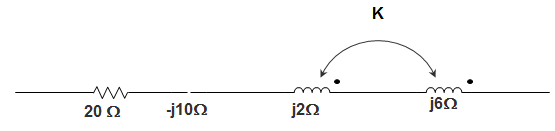Q.1
• a) 110 V
• b) 460 V
• c) 220 V
• d) 230 V
Q.2
• a) 447.2 Ω
• b) 500 Ω
• c) 235.48 Ω
• d) 325.64 Ω
Q.3
##### The impedance matrices of two, two-port network are given by [32and [5 25]. The impedance matrix of the resulting two-port network when the two networks are connected in series is ____________
• a) [3 5; 2 25]
• b) [18 7; 7 28]
• c) [15 2; 5 3]
• d) Indeterminate
Q.4
• a) 2.67 kWh
• b) 3 kWh
• c) 2.5 kWh
• d) 3.5 kWh
Q.5
##### The current rating of a cable depends on ___________
• a) Length of the cable
• b) Diameter of the cable
• c) Both length and diameter of the cable
• d) Neither length nor diameter of the cable
Q.6
• a) 2 and 0
• b) 1 and 0
• c) 0 and -1
• d) 1 and -1
Q.7
• a) 0.25
• b) 0.5
• c) 0.1
• d) 1
Q.8
##### In an RC series circuit R =Ω and XC =Ω. Which of the following is possible?• a) The current and voltage are in phase
• d) The current lags the voltage by about 6°
Q.9
##### The impedance of an RC series circuit isΩ at f =Hz. At f =Hz, the impedance will be?
• a) More than 12
• b) Less than 3
• c) More than 3 Ω but less than 12 Ω
• d) More than 12 Ω but less than 24 Ω
Q.10
##### A 3 phase balanced supply feeds a 3-phase unbalanced load. Power supplied to the load can be measured by ___________
• a) 2 Wattmeter and 1 Wattmeter
• b) 2 Wattmeter and 3 Wattmeter
• c) 2 Wattmeter and 2 Wattmeter
• d) Only 3 Wattmeter
Q.11
##### A series RC circuit has R =Ω and C = 1 μF. The current in the circuit is 5 sin 10t. The applied voltage is _____________
• a) 218200 cos (10t – 89.99°)
• b) 218200 sin (10t – 89.99°)
• c) 218200 sin (10t)
• d) 218200 cos (10t)
Q.12
• a) 0.75 F
• b) 0.75 μF
• c) 0.03 F
• d) 0.03 μF
Q.13
• a) 100 Ω
• b) 50 Ω
• c) 25 Ω
• d) 200 Ω